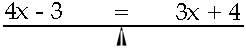Name: heather Who is asking: Student Level: Middle Question: 4x-3 = 3x+4 these kinds of problems i dont get but everything else in my math class i have got. i just dont understand it. thanks heather Hi Heather,With this problem you start with the equation 4x - 3 = 3x + 4 and you need to progress through a sequence of legitimate steps and end with an equation x = some number The crutial pattern here is that you want x on the left of the equal sign and a number on the right. It sometimes helps to think of the equation as a balance with the balance point being the equal sign. Legimate steps are steps that don't upset the balance, that is what you do to one side you must do to the other.First I would eliminate the term containing x from the right side. This can be done by subtracting 3x from the right side. To maintain the balance you need also to subtract 3x from the left side. Thus 4x - 3 = 3x + 4 becomes 4x - 3 - 3x = 3x + 4 - 3x which simplifies to x - 3 = 4 This is close to the correct form, all that remains is to eliminate the -3 from the left side. This can be done by adding 3 to the left side, and to maintain the balance, adding 3 to the right side. Thus x - 3 = 4 becomes x - 3 + 3 = 4 + 3 which is x = 7 You can verify that this is the correct answer by substituting x = 7 into the original equation, 4x - 3 = 3x + 4, to see that you get the same value on each side. Cheers, Penny Go to Math Central